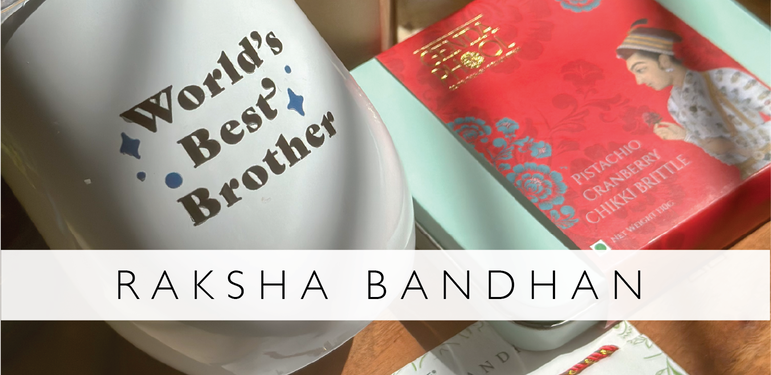# Rakhifrom Rs. 329.00

from Rs. 329.00

from Rs. 229.00

Rs. 329.00

Rs. 149.00

Rs. 149.00

Rs. 329.00

from Rs. 499.00

from Rs. 249.00

from Rs. 199.00

from Rs. 329.00

from Rs. 329.00

from Rs. 329.00

Rs. 699.00

Rs. 699.00

from Rs. 199.00

from Rs. 329.00

from Rs. 229.00

from Rs. 229.00

from Rs. 329.00

from Rs. 329.00

from Rs. 329.00

from Rs. 229.00

from Rs. 199.00

from Rs. 199.00

from Rs. 199.00

from Rs. 329.00

from Rs. 329.00

Rs. 1,260.00

Rs. 1,125.00

Rs. 2,300.00

Rs. 235.00

Rs. 250.00

Rs. 250.00

Rs. 300.00

Rs. 325.00

Rs. 250.00

Rs. 4,200.00

from Rs. 499.00

from Rs. 499.00